﻿ 波浪作用下船舶横向振动控制系统
 舰船科学技术2022, Vol. 44Issue (19): 28-31    DOI: 10.3404/j.issn.1672-7649.2022.19.006PDF

Ship transverse vibration control system under wave action
WANG Mei-yu
Weihai Ocean Vocational College, Weihai 264300, China
Abstract: The rolling vibration of a ship under the action of waves will not only reduce the working accuracy of the ship's precision equipment, but also cause the fatigue load generated by the vibration to act on the hull structure for a long time, which may also lead to the fatigue failure of the structure. Therefore, in order to ensure the safety of the ship's operation, this paper develops a ship's lateral vibration control system under the action of waves in combination with the fin stabilizer technology. The working principle and performance simulation of fin stabilizer control system are studied.
Key words: fin stabilizer     lateral vibration     hydraulic pressure     simulation
0 引　言

1 波浪作用下的船舶横向振动边界条件与船舶运动建模

 $\xi (t) = \sum\limits_{i = 1}^n {{\xi _0}(t)\cos ({k_1}\delta + {w_0}t + \varphi )} \text{。}$

 $\eta ({\omega _0}) = \frac{{{k_1}}}{{{\omega _0}^3}}\exp \left(\frac{{ - {k_2}}}{{{\omega _0}^4}}\right) \text{。}$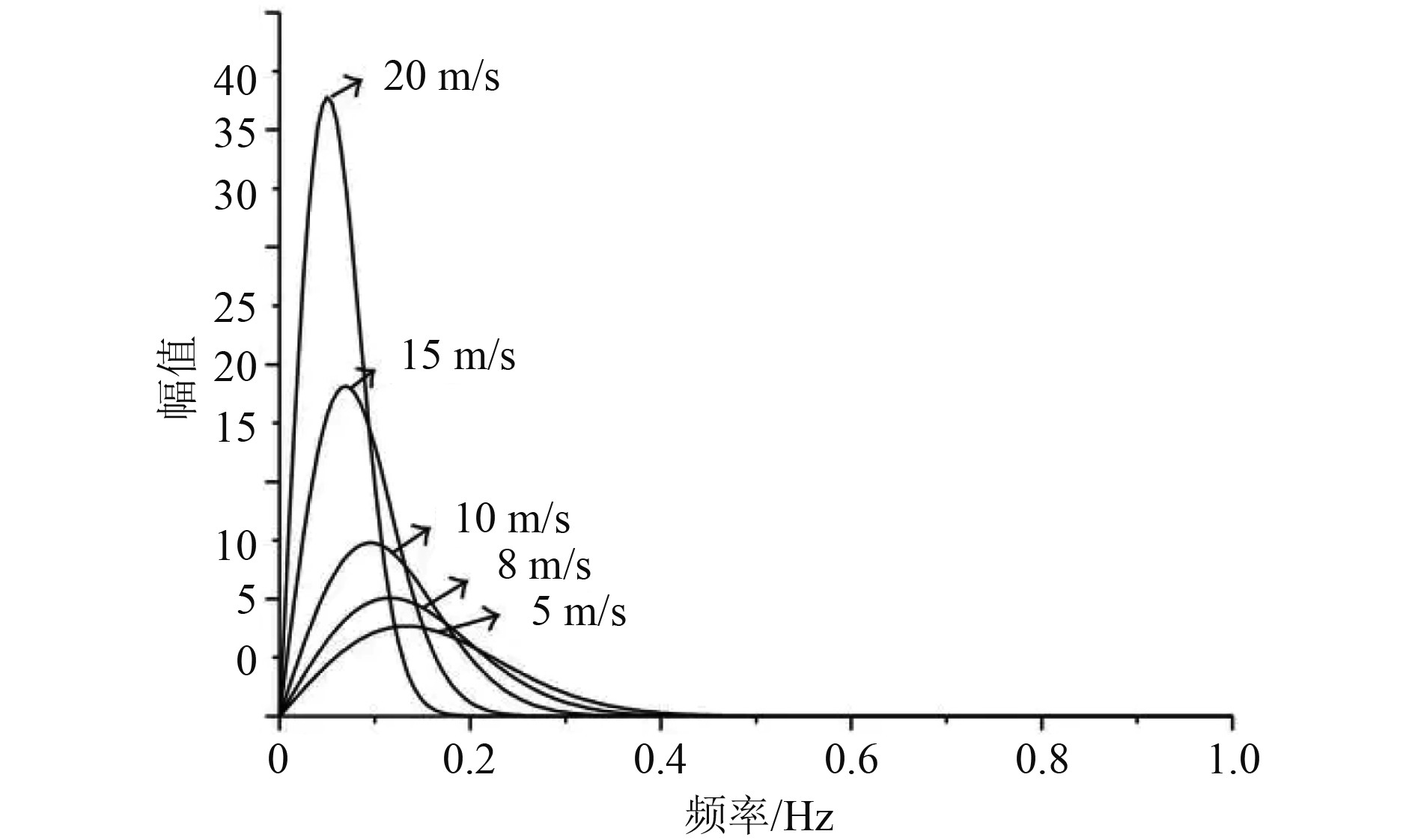图 1 波浪在不同传输速度下的幅频特性曲线 Fig. 1 Wave amplitude frequency characteristic curve at different transmission speeds

1）流体动力学边界

 $\frac{{\partial \phi }}{{\partial t}} + \frac{1}{2}\nabla {\phi ^{^2}} + g\delta = 0 。$

2）运动学边界

 $\frac{F}{M} = \frac{{\rm{d}}}{{{\rm{d}}t}}f\left[ {x,y,z} \right] \text{。}$

 $\frac{{{\rm{d}}F}}{{{\rm{d}}t}} = \frac{{\partial F}}{{\partial t}}\frac{{{\rm{d}}z}}{{{\rm{d}}t}} + \frac{{\partial F}}{{\partial t}}\frac{{{\rm{d}}y}}{{{\rm{d}}t}} + \frac{{\partial F}}{{\partial t}}\frac{{{\rm{d}}x}}{{{\rm{d}}t}} = 0 。$

3）根据船舶在波浪条件下的力学特性，建立船舶横向振动运动模型如下式：

 $\left\{ {\begin{array}{*{20}{c}} {\left( {{J_{}} + \Delta {J_{}}} \right)w + 2\kappa w + \dfrac{1}{2}Dh\theta = {T_0}}，\\ {M\dfrac{{{\rm{d}}{u_i}}}{{{\rm{d}}t}}\sin \theta + M\dfrac{{{\rm{d}}{u_j}}}{{{\rm{d}}t}}\cos \theta = {F_0}}。\end{array}} \right.$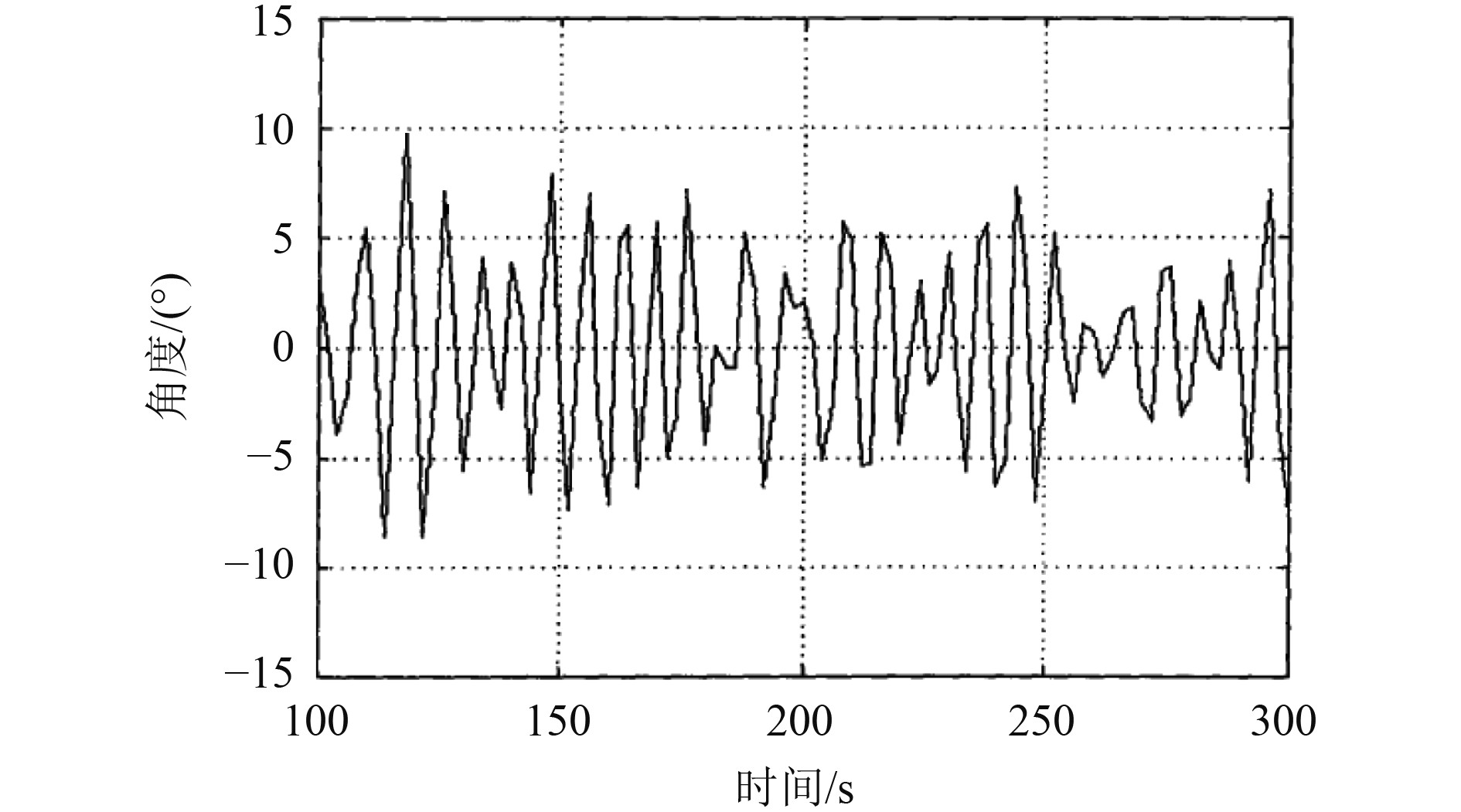图 2 船舶横向振动角度曲线图 Fig. 2 Curve of ship transverse vibration angle
2 基于减摇鳍的船舶横向振动控制系统设计及仿真 2.1 减摇鳍流体力学的基本原理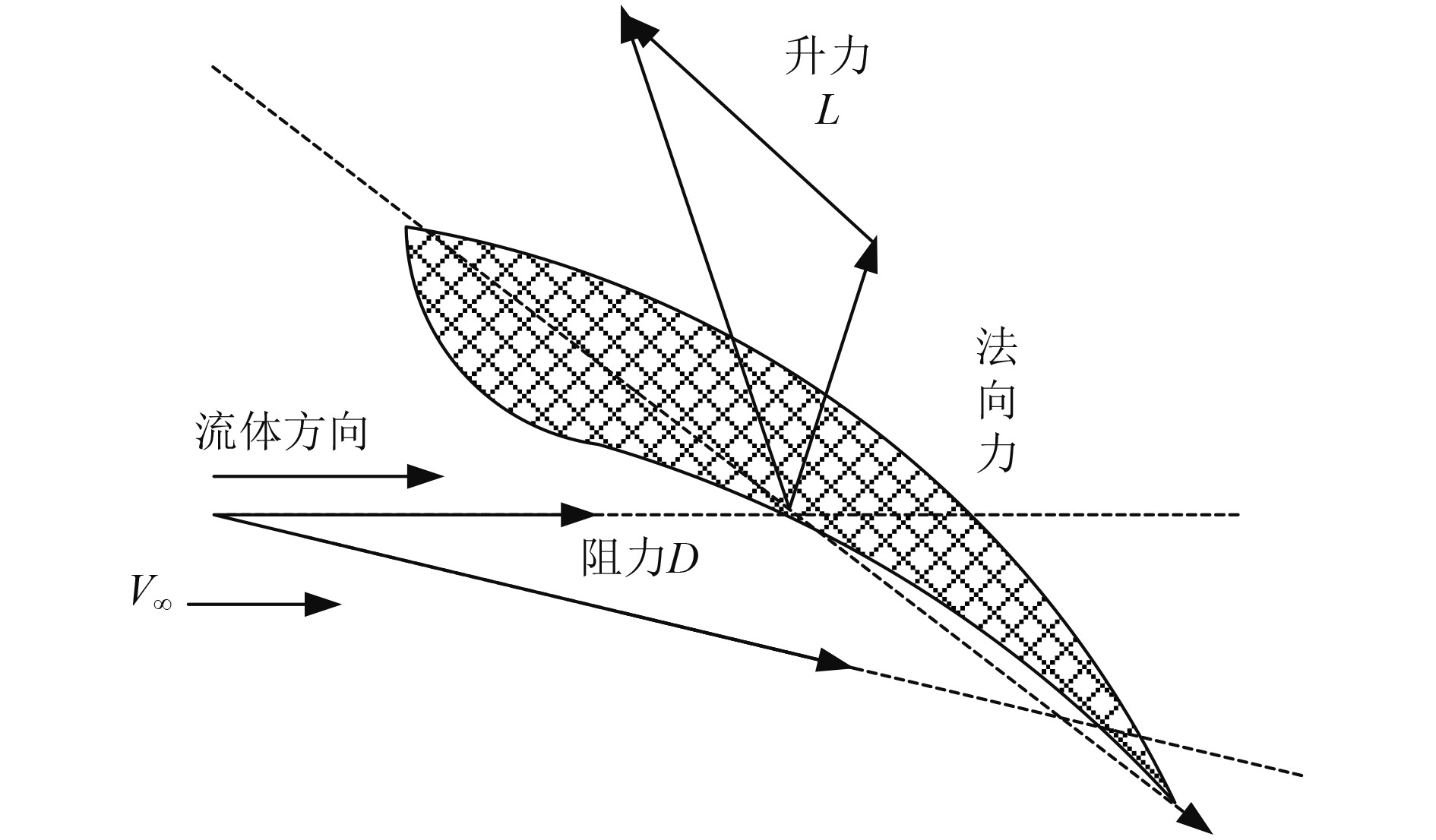图 3 减摇鳍翼型设计特征示意图 Fig. 3 Schematic Diagram of Fin Stabilizer Airfoil Design Features

1）升力系数

 ${C_L} = \dfrac{L}{{\dfrac{1}{2}\rho V_\infty ^2c}} \text{。}$

2）阻力系数

 ${C_D} = \dfrac{D}{{\dfrac{1}{2}\rho V_\infty ^2c}} \text{。}$

3）压强系数

 ${C_p} = \dfrac{{P - {P_\infty }}}{{\dfrac{1}{2}\rho V_\infty ^2}} \text{。}$

 $- {F_0} = {F_k} = \dfrac{1}{2}{\rho _{}}S \cdot {C_L}{V_\infty }^2 \text{。}$

 $- {T_0} = {T_s} = \dfrac{1}{2}h\sin \theta {F_k}\cos \beta \text{。}$表 1 减摇鳍设计参数 Tab.1 Design parameters of fin stabilizer
2.2 减摇鳍鳍型变换液压系统设计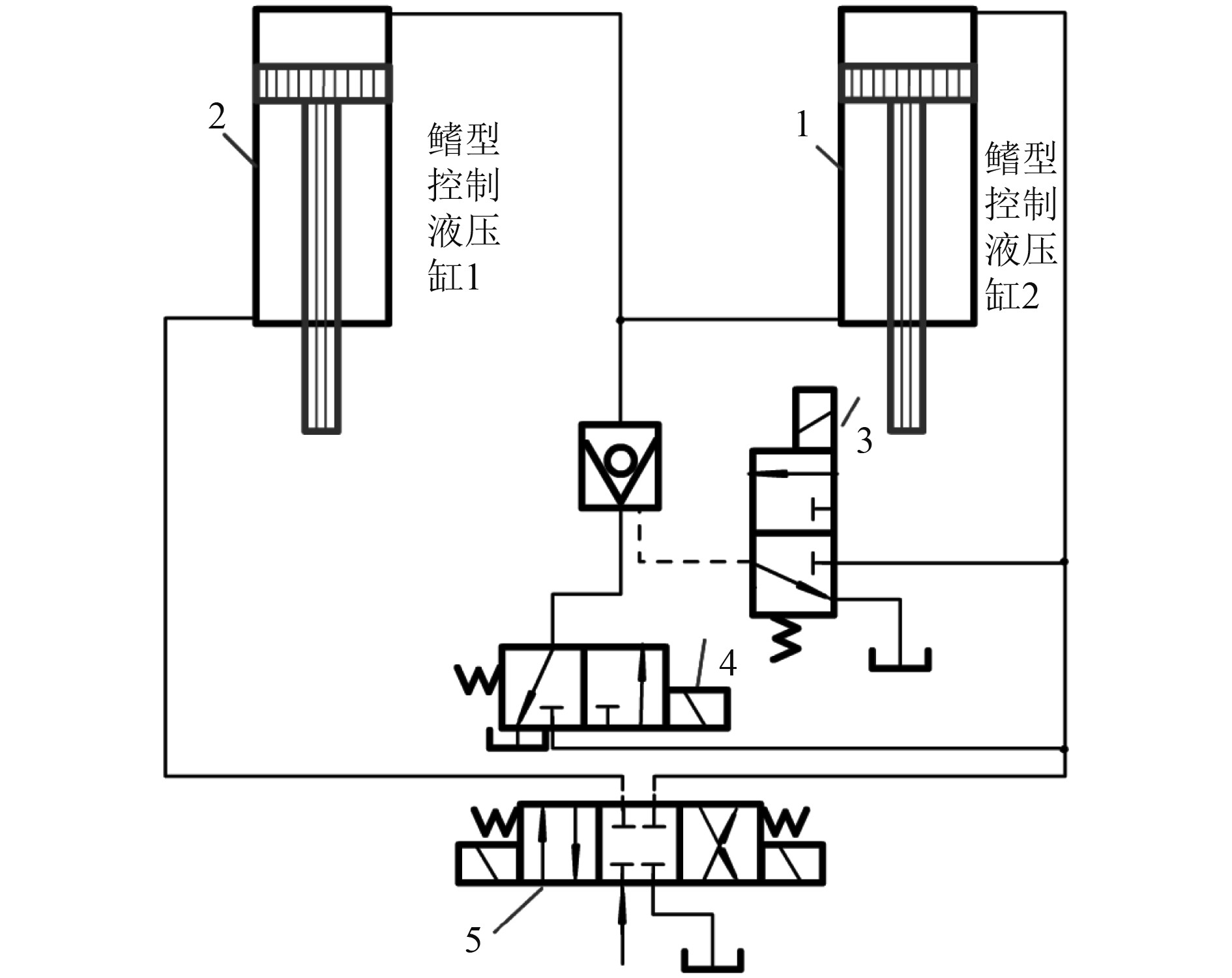图 4 减摇鳍液压系统回路原理图 Fig. 4 Circuit schematic diagram of fin stabilizer hydraulic system

2个液压缸分别驱动船舶两侧减摇鳍，实现鳍型的变化，根据波浪作用力的大小调节恢复力矩的大小，横向运动控制力矩与液压回路的控制模型如下式：

 ${M_{\text{Z}}} = \dfrac{{\text{π} D_d^2p{R_0}{\eta _m}}}{{4{{\cos }^2}\alpha }} \text{。}$

2.3 波浪作用下船舶横向振动控制系统设计

 $f(t) = Ax\left( t \right) + By\left( t \right) \text{，}$

 $\left\{ {\begin{array}{*{20}{c}} {{{\dot f}_1}(t) = {A_1}{x_1}\left( t \right) + {B_1}{y_1}\left( t \right)}，\\ {{{\dot f}_2}(t) = {A_2}{x_2}\left( t \right) + {B_2}{y_2}\left( t \right)}，\\ {...} \\ {{{\dot f}_n}(t) = {A_n}{x_n}\left( t \right) + {B_n}{y_n}\left( t \right)} 。\end{array}} \right.$

 $S(x,t) = Cx(t) = 0 \text{，}$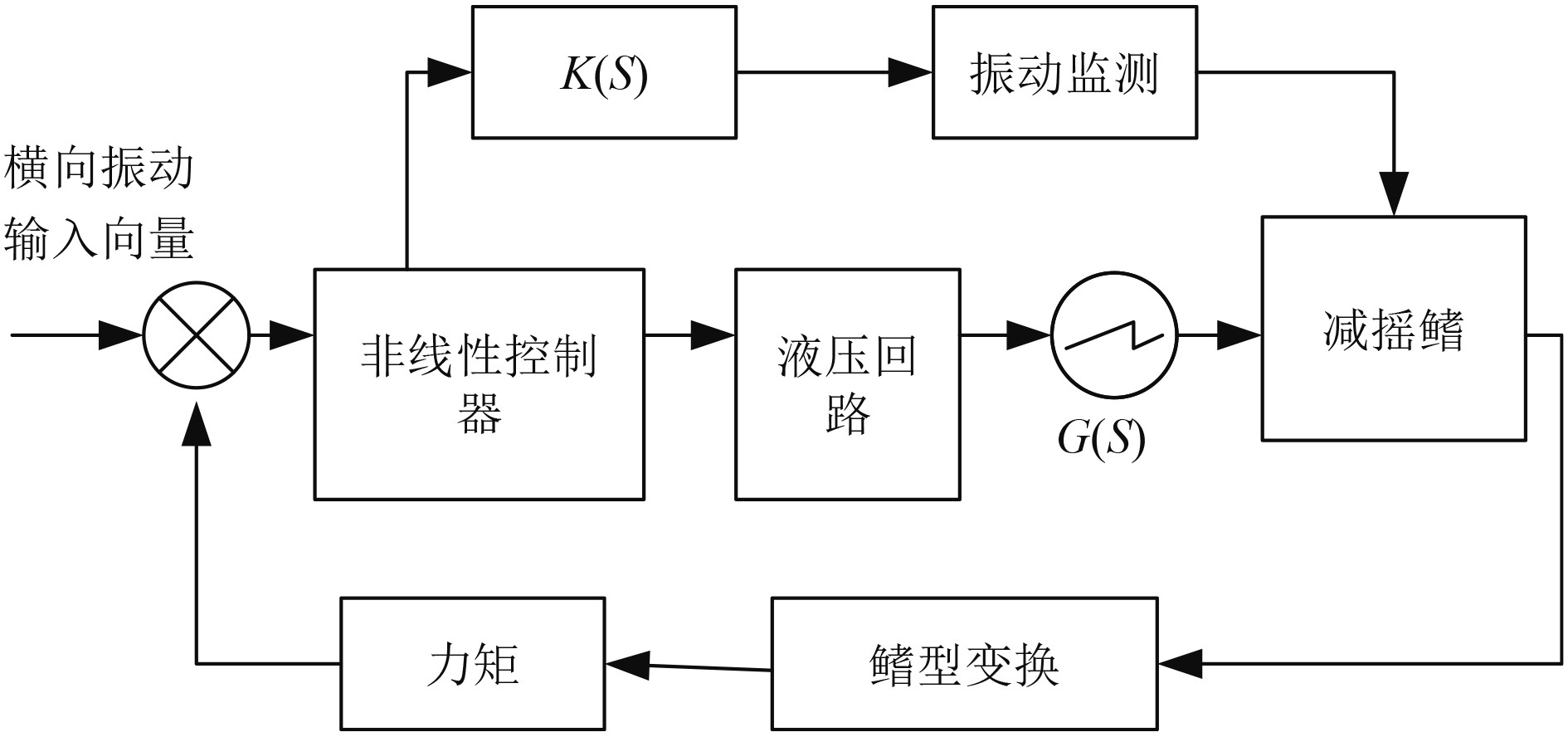图 5 船舶横向振动控制系统原理图 Fig. 5 Schematic diagram of ship transverse vibration control system

 $G(s) = {k_1} + \frac{{{k_2}}}{\omega } + \frac{{{k_3}}}{{{\omega ^2}}}{s^2} \text{。}$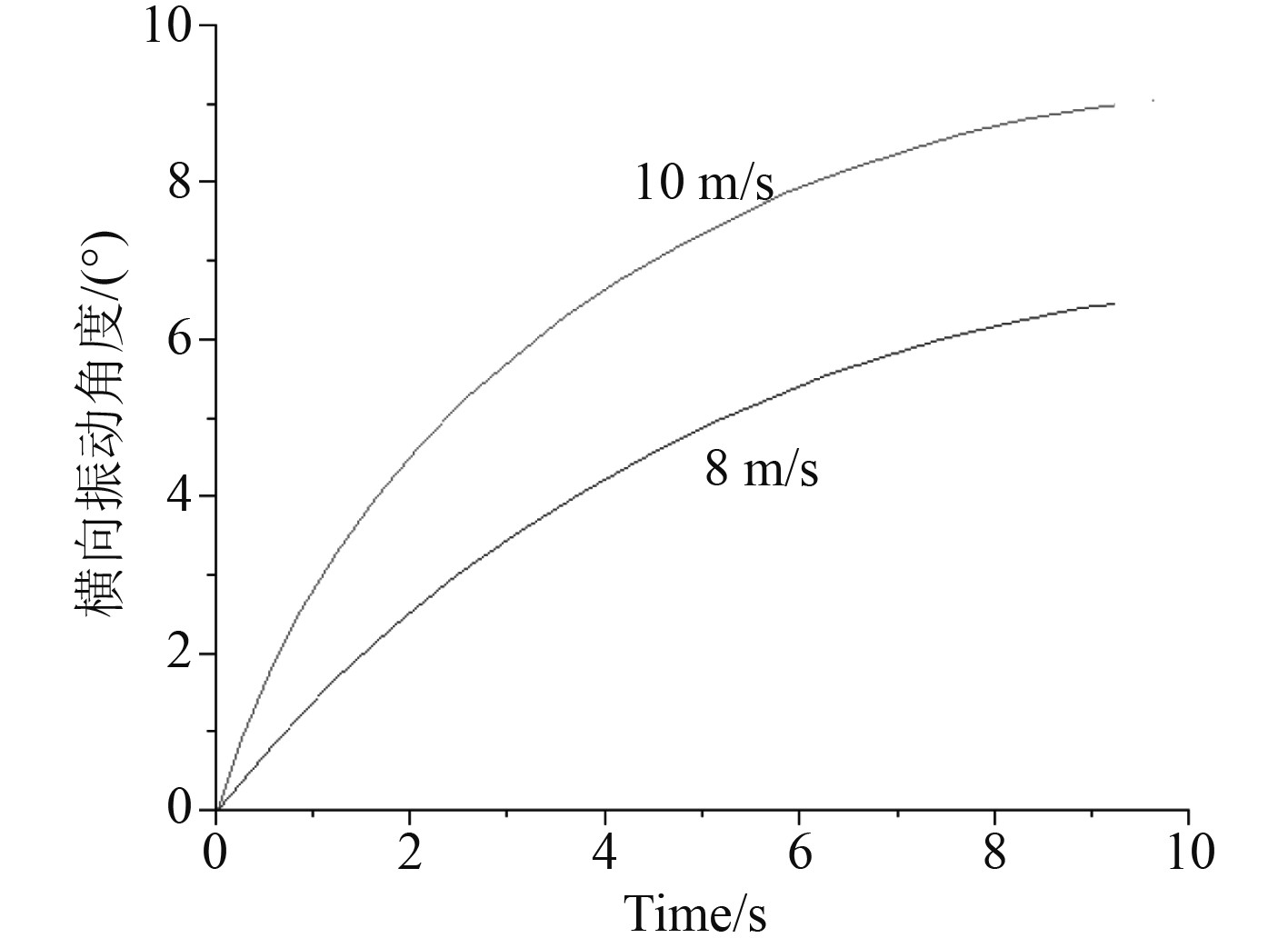图 6 横向振动角度控制曲线 Fig. 6 Lateral vibration angle control curve
3 结　语

  刘义. 基于陀螺减摇装置的船舶横摇减摇研究[J]. 船舶, 2022, 33(4): 124-131. LIU Yi. Research on Ship Roll Reduction Based on Gyro Stabilizer[J]. Ship, 2022, 33(4): 124-131. DOI:10.19423/j.cnki.31-1561/u.2022.04.124  任元洲. 舵鳍联合动态校正最优控制自航船模试验研究[J]. 舰船科学技术, 2022, 44(14): 52-56. REN Yuan-zhou. Self propelled ship model test research on optimal control of rudder fin joint dynamic correction[J]. Ship Science and Technology, 2022, 44(14): 52-56. DOI:10.3404/j.issn.1672-7649.2022.14.012  裴斐, 林焰. 减摇水舱性能晃荡平台试验研究[J]. 大连理工大学学报, 2022, 62(4): 349-356. PEI Fei, LIN Yan. Experimental study on sloshing platform for anti rolling tank performance[J]. Journal of Dalian University of Technology, 2022, 62(4): 349-356. DOI:10.7511/dllgxb202204003  周健. 减摇鳍装置节能控制技术优化设计[J]. 机电设备, 2022, 39(4): 156-159. ZHOU Jian. Optimal Design of Energy Saving Control Technology for Fin Stabilizer[J]. Electromechanical Equipment, 2022, 39(4): 156-159. DOI:10.16443/j.cnki.31-1420.2022.04.028